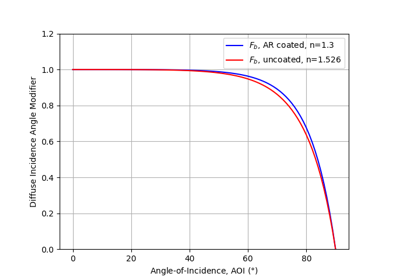# pvlib.iam.martin_ruiz#

pvlib.iam.martin_ruiz(aoi, a_r=0.16)[source]#

Determine the incidence angle modifier (IAM) using the Martin and Ruiz incident angle model.

Parameters
• aoi (numeric, degrees) – The angle of incidence between the module normal vector and the sun-beam vector in degrees.

• a_r (numeric) – The angular losses coefficient described in equation 3 of 1. This is an empirical dimensionless parameter. Values of a_r are generally on the order of 0.08 to 0.25 for flat-plate PV modules.

Returns

iam (numeric) – The incident angle modifier(s)

Notes

martin_ruiz calculates the incidence angle modifier (IAM) as described in 1. The information required is the incident angle (AOI) and the angular losses coefficient (a_r). Note that 1 has a corrigendum 2 which clarifies a mix-up of ‘alpha’s and ‘a’s in the former.

The incident angle modifier is defined as

$IAM = \frac{1 - \exp(-\frac{\cos(aoi)}{a_r})} {1 - \exp(\frac{-1}{a_r})}$

which is presented as $$AL(\alpha) = 1 - IAM$$ in equation 4 of 1, with $$\alpha$$ representing the angle of incidence AOI. Thus IAM = 1 at AOI = 0, and IAM = 0 at AOI = 90. This equation is only valid for -90 <= aoi <= 90, therefore iam is constrained to 0.0 outside this interval.

References

1(1,2,3,4)

N. Martin and J. M. Ruiz, “Calculation of the PV modules angular losses under field conditions by means of an analytical model”, Solar Energy Materials & Solar Cells, vol. 70, pp. 25-38, 2001.

2

N. Martin and J. M. Ruiz, “Corrigendum to ‘Calculation of the PV modules angular losses under field conditions by means of an analytical model’”, Solar Energy Materials & Solar Cells, vol. 110, pp. 154, 2013.

## Examples using pvlib.iam.martin_ruiz#Diffuse IAM Calculation

Diffuse IAM Calculation